# 1711 is a Triangular Number So The Taxman Wants His Share

### Today’s Puzzle:

Imagine this puzzle is made up of 58 envelopes, each containing the amount of money printed in bold type on its front. You can’t take any of the envelopes without the Taxman also taking a share. The Taxman will take EVERY available envelope that has a factor of the number you take on it. When you have taken all the cash you can, the Taxman gets ALL the leftover cash, and the game is over. You want the Taxman to get as little money as possible.

How much money is at stake? (58 × 59)/2 = 1711. That means 1711 is a triangular number, the sum of all the numbers from 1 to 58. Thus, the total amount of money you will be splitting with the Taxman is 1711.

To play this game, you can print the cards from this excel file: Taxman & 1537-1544. The factors of a number is printed in small type at the top of its card.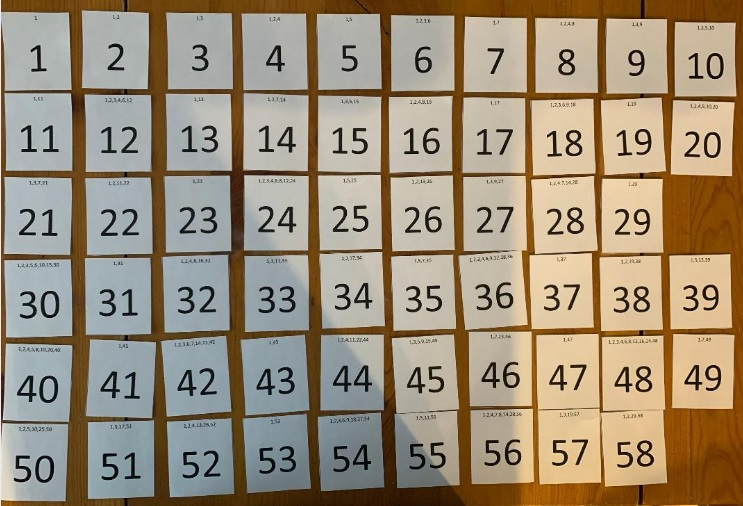Here below I show the order I selected the cards when I played. For example, I took 53, and all the Taxman got was 1. I took 49, and the only card left for the Taxman to take was 7, and so forth. The last card I could take was 42, and the Taxman got 21, but since I couldn’t take anymore cards, the Taxman also got 31, 34, 37, 41, 43, and 47.To win the game, you must get over half of the 1711 cash, but of course, you will want the Taxman to get much less than nearly half the money.

I didn’t want to make a long addition problem to find out how much I kept, so I came up with an easier strategy: I pushed the Taxman’s share to the side, and looked for ways to make 100 from my cards. (It is almost the 100th day of school, after all.) I found 9 ways to make 100, so I clearly kept more than half of the 1711.I added that final 53 + 50 + 39 + 36 in my head by thinking that if I took 3 away from 53 and gave it to the 36, it would be the same as 50 + 50 + 39 + 39, a fairly easy sum.

### Factors of 1711:

• 1711 is a composite number.
• Prime factorization: 1711 = 29 × 59.
• 1711 has no exponents greater than 1 in its prime factorization, so √1711 cannot be simplified.
• The exponents in the prime factorization are 1 and 1. Adding one to each exponent and multiplying we get (1 + 1)(1 + 1) = 2 × 2 = 4. Therefore 1711 has exactly 4 factors.
• The factors of 1711 are outlined with their factor pair partners in the graphic below.

### 1711 is a Shape-Shifting Number:

• 1711 is the 58th Triangular Number because (58·59)/2 = 1711.
• It is the 20th Centered Nonagonal Number because it is one more than nine times the 19th triangular number: 9(19·20)/2 + 1 =1711. AND
• It is the 19th Centered Decagonal Number because it is one more than 10 times the 18th triangular number: 10(18·19)/2 +1 = 1711.

That last figure I’ve illustrated below with ten triangles circled around the center dot: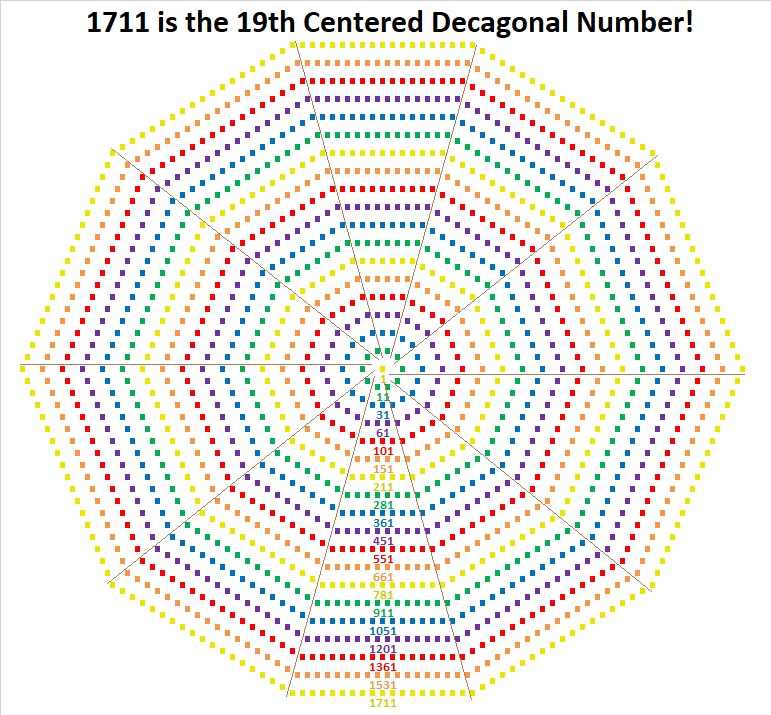Now get this: Not only is 1711 the 19th Centered Decagonal Number, but similar-looking 17111 is the 59th Centered Decagonal Number! (A mere coincidence, but the 59th is even cooler because 59·29 = 1711.)

### More About the Number 1711:

1711 is the hypotenuse of a Pythagorean triple:
1180-1239-1711, which is (20-21-29) times 59.

1711 is the difference of two squares in two different ways:
856² – 855² = 1711, and
44² – 15² = 1711.

1711 is a fun number to explore!

# How Much of My \$1596 Will the Taxman Get?

### Today’s Puzzle is a Game Called Taxman:

Tax season in the United States began just a few days ago. Figuring taxes is no fun, but playing Taxman is.

You see 56 cards below. Imagine that they are 56 envelopes, each containing the dollar amount written on them.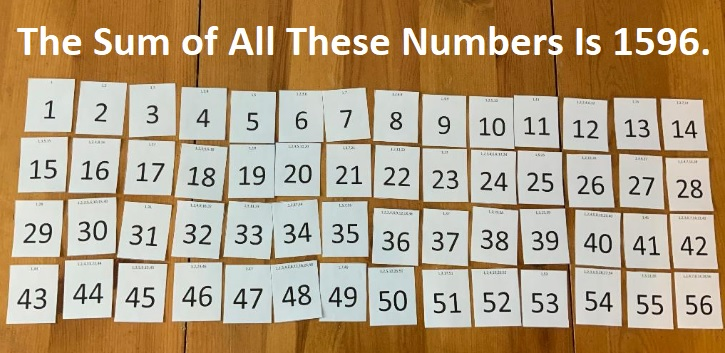You can take whatever envelope you’d like, but the taxman must be able to take at least one envelope when you do. What envelopes will the taxman take when it is his turn? He will take EVERY available envelope that has a number on it that is a factor of the envelope you took. So if you started off taking envelope 56, the taxman would take envelopes 1, 2, 4, 7, 8, 14, and 28. Thus the taxman would start off getting a whole lot more money than you while also limiting envelopes you can choose later. For example, you wouldn’t be able to pick any prime numbers after taking 56  because the 1 would be gone, and the taxman must be able to take one envelope with every choice you make. When you get to the point that there are no more envelopes that you can take, the taxman gets to take ALL the remaining envelopes. You want the taxman to get less money than you do, and hopefully as little money as possible. I’ve included the factors of each of the numbers at the top of each “envelope” to make the game easier for both you and the taxman. You can print “envelopes” from this excel file Taxman & 1537-1544.  You can have a friend be the taxman as you play or you can play both roles.

### How I Played the Game:

I took envelopes as I asked myself these questions:

What is the largest prime number on the table? 53. I take 53, and the only envelope the taxman gets is 1.

What is the largest number that is a prime number squared? 49. I take 49, and the only envelope left for the taxman to take is 7.

What is the largest multiple of 7 that has only one other available factor? 35. I take 35, and the only envelope the taxman can still get is 5.

What is the largest multiple of 5 that has only one other available factor? and so forth.

Here is the order I took the envelopes. It is not the only possible order to use, but it was one in which there were only nine cards at the end of the game for the taxman to claim.The winner of the game is the one with the most money at the end of the game.

### How Do We Know Who Won the Game?

We could add up all the money I got and compare it with all the money the taxman got, but that wouldn’t be much fun. We could add up all the taxman money and subtract it from 1596 to find my total, but I prefer a different way: I remove all the taxman’s money from the table and try to mix envelopes to match mine. I start with my envelope with the most cash, 56 = 8 + 1 + 47, so I put the taxman’s envelopes with those numbers next to my 56.

Matching the “envelopes” up like this will take some time, but it feels like playing a game. It may have taken me as long to play this part of the game as it took me to play Taxman because sometimes I had to remove perfectly good sums in order to use all the taxman’s envelopes in a sum. Here is how I matched the envelopes:I know that I won the game because I had at least one envelope without a sum next to it. I can determine by  how much I won the game by adding 35 + 33 + 46 + 39 + 27 + 38 + 20 + 30 + 42. That sum tells me I won the game by \$310.

How much did the taxman get?
(1596-310)/2 = 643.

How much did I get to keep?
643 + 310 = 953.

### A Factor Tree for 1596:

If you know that 40² = 1600, and 1600 – 4 = 1596, we might recognize that 1596 is the difference of two perfect squares: 40² – 2². Then we can also know that
1596 = (40 – 2)(40 + 2) = 38 × 42.

Let’s make a factor tree from the factor pair 38 × 42: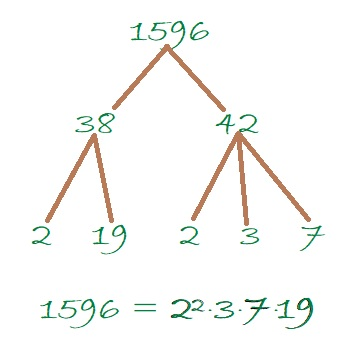Since 1596 has several factors, it has several other possible factor trees.

### Factors of 1596:

• 1596 is a composite number.
• Prime factorization: 1596 = 2 × 2 × 3 × 7 × 19, which can be written 1596 = 2² × 3 × 7 × 19.
• 1596 has at least one exponent greater than 1 in its prime factorization so √1596 can be simplified. Taking the factor pair from the factor pair table below with the largest square number factor, we get √1596 = (√4)(√399) = 2√399
• The exponents in the prime factorization are 2, 1, 1, and 1. Adding one to each exponent and multiplying we get (2 + 1)(1 + 1)(1 + 1)(1 + 1) = 3 × 2 × 2 × 2 = 24. Therefore 1596 has exactly 24 factors.
• The factors of 1596 are outlined with their factor pair partners in the graphic below.### More about the Number 1596:

The reason that I was dividing up \$1596 between me and the taxman is that 1596 is the 56th triangular number. It is the 56th triangular number because
(56)(57)/2 = 1596.

1596 is the difference of two squares in four different ways:

1596 is the sum of the first 15 Fibonacci numbers, which also means it is one number less than the 17th Fibonacci number.# How Much of My \$1540 Will The Taxman Get?

### The Taxman Game

You might think that the picture below is just the numbers from 1 to 55 arranged in a 5×11 rectangle.

It’s really PLAY MONEY, one bill in each denomination from 1 to 55. If you add up the total value of all this play money, you will get 1540 because the sum of the numbers from 1 to 55 is 1540.Most of that 1540 in play money can be yours to keep if you outsmart THE TAXMAN.

You can take any bill that you want, but The Taxman has to get paid every time you do. The Taxman will get ALL the available factors of the bill you take. If there isn’t at least one bill on hand that is a factor of your chosen bill, then you can’t take that bill. When none of the bills left are factors of any of the other bills that are left, The Taxman gets ALL the remaining bills. The value of those bills could add up quickly, and that’s how The Taxman might outsmart you.

You can print this excel file to play the Taxman game: Taxman & 1537-1544 It has all the play money bills from 1 to 100, and all the factors are typed on the top of the bills!

The factors at the top of each bill make it possible to play the game even if you don’t know how to multiply or divide yet. My granddaughter learned to play the game sometime around her eighth birthday. Before tackling 55 bills, she learned what to do for a smaller number of bills. I told her:
If 1 is the only bill, The Taxman wins.
If 1, 2 are the only bills, you take the 2, The Taxman gets the 1, and you win.
If 1, 2, 3 are the only bills, the best you can do is a draw (1 + 2 = 3),
But if there are at least four bills, it is possible to win every time.

She played the game over and over adding one more bill to the game each time she played. She enjoyed it very much and played it over 20 times until bedtime required her to stop. Each time she played we talked about how she would arrange the cards in a rectangle. For example, if she used cards 1 – 6, she would look at the factors of 6 and decide on a 2 × 3 rectangle or a 1 × 6 rectangle.

Knowing I wanted to write this post about the 55th triangular number (1540), I had her jump to using 55 bills the next day, but I helped her with it a little. We didn’t want to just win the game, we wanted to make it so The Taxman would get as little money as possible. Here is the final order of what we took and what The Taxman got.Laying it out like that made it easy to have do-overs when needed.

### A Way to Keep Score When Playing Taxman:

When it was time to score the game, instead of adding up all the numbers to see if we took more than The Taxman, we found smaller sums that equaled as many of our choices as we could as illustrated below. For example,  47 + 6 = 53, so there was no need to add 47 + 6 to The Taxman’s total or to add 53 to ours.I found it enjoyable finding all those sums, but it isn’t necessarily easy for a child to do. After arranging all those sums on the table, we were able to see that we scored
35 + 39 + 38 + 12 + 30 + 50 + 24 + 48 + 42 = 318 more than The Taxman did.

We can use algebra to figure out exactly how much of our 1540 in play money we get to keep.
If The Taxman got X of the play money, we kept X + 318 of it, and
X + X + 318 = 1540,
2X + 318 = 1540,
2X + 318 – 318 = 1540 – 318,
2X = 1222,
X = 611, that’s what The Taxman got.
We kept 611 + 318 = 929 of our play money.

What percentage of the play money did The Taxman get?
611/1540 ≈ 0.40 which is 40%. Wow, that’s a lot!

### Factors of 1540:

• 1540 is a composite number.
• Prime factorization: 1540 = 2 × 2 × 5 × 7 × 11, which can be written 1540 = 2² × 5 × 7 × 11.
• 1540 has at least one exponent greater than 1 in its prime factorization so √1540 can be simplified. Taking the factor pair from the factor pair table below with the largest square number factor, we get √1540 = (√4)(√385) = 2√385.
• The exponents in the prime factorization are 2, 1, 1, and 1. Adding one to each exponent and multiplying we get (2 + 1)(1 + 1)(1 + 1)(1 + 1) = 3 × 2 × 2 × 2 = 24. Therefore 1540 has exactly 24 factors.
• The factors of 1540 are outlined with their factor pair partners in the graphic below.### Factor Cake for 1540:

1540 has lots of prime factors with 11 being the greatest so it makes a lovely factor cake:### What Kind of Shape Is 1540 in?

I know that 1540 = 55×56÷2 so, 1540 is the 55th triangular number. Guess what? Since 55 is also a triangular number (55 = 10×11÷2), we could have arranged the play money bills in a triangle like this instead of a 5 × 11 rectangle.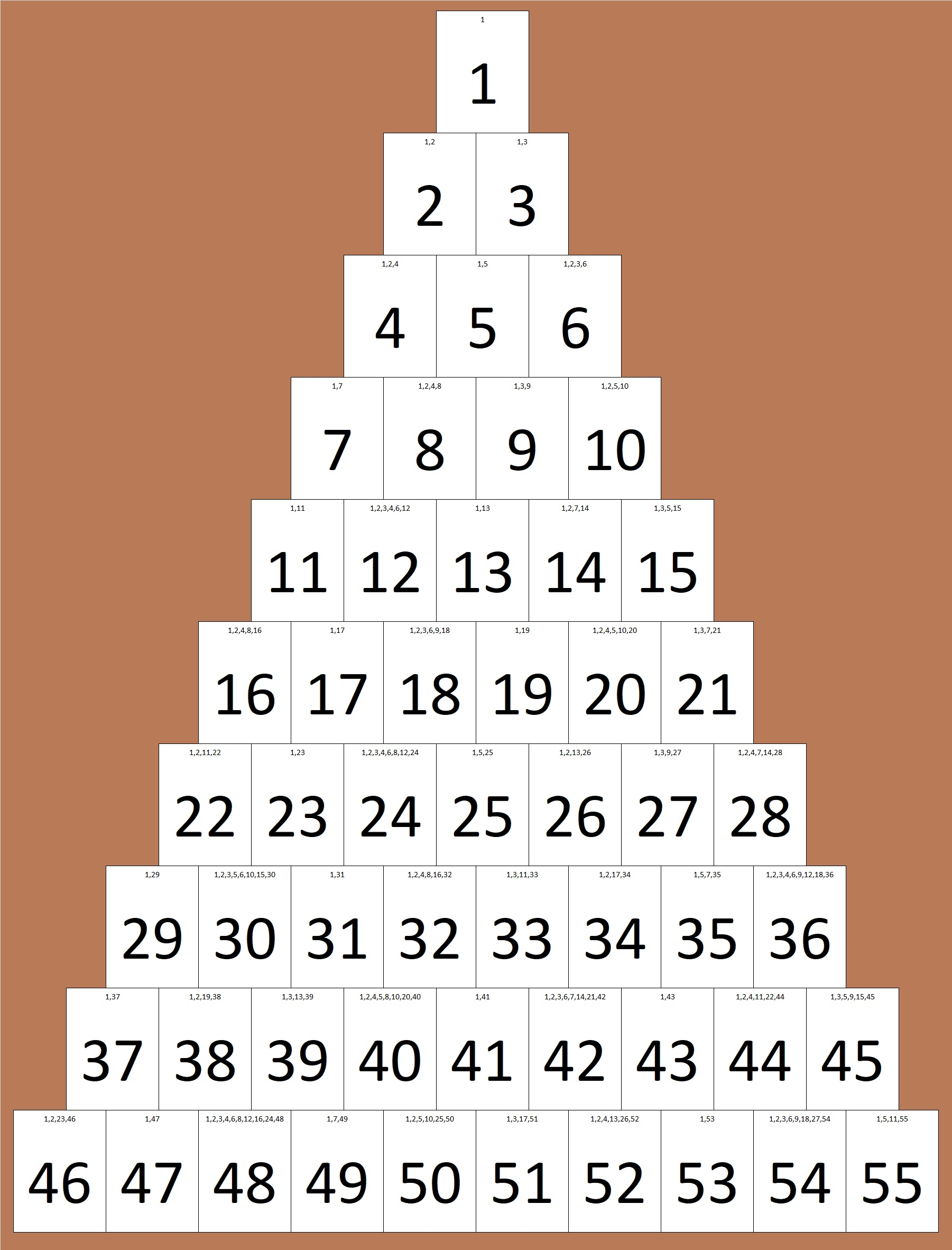1540 is not only the 55th triangular number, but it is also the sum of the first 20 triangular numbers:

1+3+6+10+15+21+28+36+45+55+66+78+91+105+120+136+153+171+190+210 = 1540.

If you stack those twenty triangular numbers from smallest to biggest, you will get something shaped like a tetrahedron. That’s why we say that 1540 is the 20th tetrahedral number. We can also use a formula for the 20th tetrahedral number:
20(21)(22)/6 = 1540.

1540 is also the 28th hexagonal number. (All hexagonal numbers are also triangular numbers. That’s why it’s the 2(28)-1 = 55th triangular number.) It is the 28th hexagonal number because 2(28²) – 28 = 1540.1540 is the 20th decagonal number because 4(20²) – 3(20) = 1540.I didn’t make a visual, but 1540 is also the19th centered nonagonal number.
That’s because
3 × 19 = 57 and (57 – 2)(57 – 1)/2 = 1540.

1540 is also the hypotenuse of a Pythagorean triple triangle:
924-1232-1540 which is (3-4-5) times 308.

1540 is quite the shape-shifter number, isn’t it!